Home MonkeyNotes Printable Notes Digital Library Study Guides Study Smart Parents Tips College Planning Test Prep Fun Zone Help / FAQ How to Cite New Title Request

1.4 Real Numbers

It was only in the last quarter of 19th century that three proper theories of the structure of real numbers were separately proposed by three German mathematicians viz., K. Weirestrass, R Dedekind and G. Cantor.

By the axioms formulated by G. Peano (1858 - 1932), the set of natural numbers (N) is completely defined.

In order to make subtraction possible, the set I of integers is defined.

Again to make division possible, the set Q of rational numbers is then defined.

To fill up the gaps in certain representations or classifications, the concept of irrational numbers is introduced. The totality of the rational and irrational numbers is the set of real numbers R.

The rational numbers

If p, q are integers, q ą 0 then the number denoted by p/q with the property p/q ´ q = q ´

p/q = p is called the rational number. Thus Q =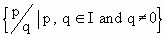Note

(1) In the definition of Q, the condition q ą 0 is necessary since division by zero is not permitted.

(2) Integers are also rational because every integer p = p/1. Hence I Í Q

Equality Relation on Q : P/q and r / s with q, s ą 0 are two rational numbers, then

p/q = r/s if and only if ps = qr.

Order Relation on Q : When q, s > 0, p/q < r/s if and only if ps < qr. Except for division by ’O’, Q is closed under operations of addition, subtraction, multiplication and division.Properties of rational numbers

If a, b, c are any rational numbers, then

1. a + b = b + a

2. (a + b) + c = a + (b + c)

3. The rational number O has property a + 0 = 0 + a = a.

4. For every 'a' there exists '-a' such that a + (- a) = (- a) + a = 0

5. a. b = b . a

6. (a . b) . c = a . (b . c)

7. The rational number 1 has the property that a . 1 = 1. a = a

8. For each rational number a(a ą 0), there exists a rational number 1/a such that

a . 1/a = 1/a . a = 1

9. a . (b + c) = ab + ac and (a + b) c = ac + bc

Representation of rational numbers in decimal form

Any positive rational number p/q, after actual division, if necessary can be expressed as,

p / q = m + r/q where m is non-negative integer and 0 Ł r < q

For example ,For decimal representation of p/q, now we have merely to consider the decimal form of fraction r/q which we usually write to the right of the decimal point.

Consider some fractions given below.

(1)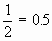(2)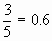(3)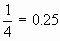(4)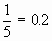(5)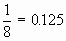(6)(7)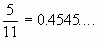(8)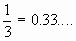(9)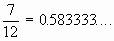Note that the dots ........ represent endless recurrence of digits.

Examples (1), (2), (3), (4) and (5) suggest that we have decimal form of the "terminating type". While examples (6), (7), (8) and (9) tell us that we have decimal form of the "non-terminating type".

In case of "non-terminating type" we have decimal fractions having an infinite number of digits. Some decimal fractions from this group have digits repeating infinitely. They are called "repeating or recurring " decimals.

In "endless recurring or infinite repeating" decimal fractions we can see that when p is actually divided by q the possible remainders are 1, 2, 3, ..... , q - 1. So one of them has to repeat itself in q steps. Thereafter the earlier numeral or group of numerals must repeat itself.

Note

(1) All the rational numbers thus can be represented as a finite decimal (terminating type) or as a recurring decimal.

(2) The recurring digits from the recurring group are indicated by putting a dot above

the first and last of them or a bar above the recurring group.

For example (i) 0.333.......as 0.3 or 0.3 (ii) 1.2555 .... as 1.25 or 1.25

(iii) 3.142142142 ..... As 3.142 or 3.142

* The line segment or bar is called a ’vinculum’

* Every infinite repeating decimal can be expressed as a fraction.

Index

Introduction
1.1 Pre-requisties
1.2 Common Mathematical Symbols
1.3 Some Properties of Basic Mathematical Operations
1.4 Real Numbers

Chapter 2Search: All Products Books Popular Music Classical Music Video DVD Toys & Games Electronics Software Tools & Hardware Outdoor Living Kitchen & Housewares Camera & Photo Cell Phones Keywords: